# Polyhedra - PuzzlesHappy Cube Six-Pack \$19.95 A classic, created in 1986 by Belgian inventor Dirk Laureysens, this puzzle is simple yet challenging. There are six pieces in each foam color, each group of six different, and each forming a cube when assembled in the right order. In addition, there are many other brain teasers using the same pieces. A personal favorite of MathArtFun founder Robert Fathauer. Ages 5 and up.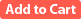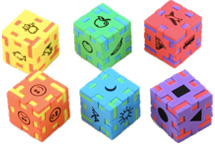Little Genius Cube Six-Pack \$19.95 This version of the Happy Cube is a little easier, for children ages 3-7.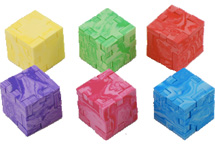Marble Cube Six-Pack \$19.95 This version of the Happy Cube is extra challenging, for ages 9 and up.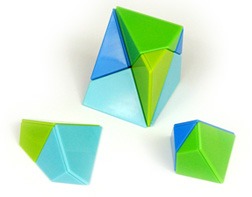Pentadron \$34.95 Pentadron consists of twelve plastic pieces with magnets inside that only allow them to fit together certain ways. The twelve pieces are all shaped the same, except for the fact that half are mirror images of the others. Assembling all twelve pieces into a cube is a challenging puzzle. This remarkable shape, a recent discovery by Jin Akiyama, is an elementary building block that allows construction of all five of Federov's parallel polyhedra. These space-filling polyhedra are the cube, the hexagonal prism, the rhombic dodecahedron, the elongated rhombic dodecahedron, and the truncated octahedron. (The four other than the cube take more than 12 pieces.) Ages 8 to adult.The Irrational Puzzle \$19.95 This set contains four cubes dissected four different ways to form a variety of geometric solids. The instructions contain seven brain teasers in which different three-dimensional geometric shapes are formed with the pieces. This puzzle makes use of the fact that the face diagonal and space diagonal of a unit cube are the squares roots of 2 and 3, respectively, to introduce students to the concept of irrational numbers. Three reproducible worksheets cover the history of the square root of 2, the discovery of irrational numbers, and the Pythagorean Theorem. Contains 11 geometric solids made from soft, durable, and nontoxic foam. Cubes measure 2.5" on a side. Grades 3-12.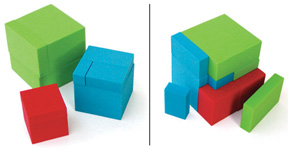Cubed Cubes \$14.95 Cubed Cubes is a brain teaser puzzle that teaches students about rectangular solids and the cubic numbers. The foam pieces form red, blue, and green cubes, each of a different size. In addition, all nine pieces together form a larger cube. The puzzle is based on the mathematical curiosity that the cubes of 3, 4 and 5 sum to the cube of 6. The instructions include several additional three-dimensional puzzles to solve using the same pieces. Also included are classroom worksheets on the volume of rectangular solids and cubic numbers. Grades 2-12.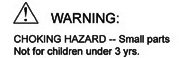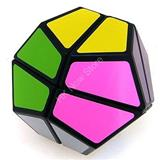Small Dodecahedron Twisty \$9.95 A simpler twisty puzzle based on the regular dodecahedron. This is a visually-appealing design, but relatively easy to solve.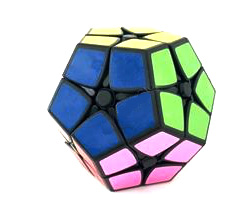Dodecahedron "2 x 2" Twisty Puzzle \$12.95 This "2 x 2" twisty puzzle is in the form of a regular dodecahedron.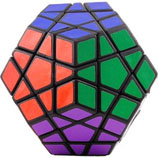Megaminx Dodecahedron Puzzle \$19.95 The 12 different colors of this high-quality rotational puzzle should throw you for a loop as to the solution. On a scale of 1-10, this puzzle rates a 10 for difficulty. 3 1/4 in x 3 1/4 in x 2 2/3 in.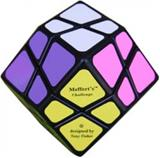Rhombic Dodecahedron Twisty Puzzle \$14.95 This twisty puzzle is in the form of a rhombic dodecahedron (twelve faces, each of which is a rhombus).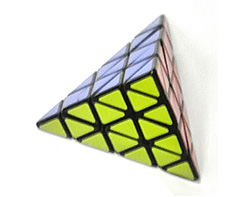Pyraminx Puzzle \$19.95 This "3 x 3" twisting puzzle is shaped like a regular tetrahedron, or triangular pyramid. Measures 3.7" x 3.2" x 3.2".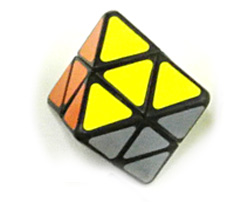Octahedron "2 x 2" Twisty Puzzle \$9.95 This "2 x 2" twisty puzzle is in the form of a regular octahedron (eight faces, each of which is an equilateral triangle).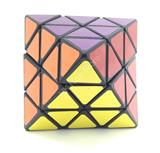Octahedron Twisty Puzzle \$14.95 This twisty puzzle is in the form of a regular octahedron (eight faces, each of which is an equilateral triangle).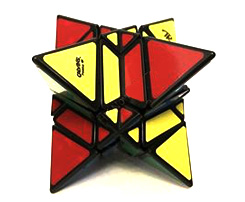Stella Octangula Twisty Puzzle \$19.95 This twisty puzzle is in the form of a stella octangula, which is same as two interpenetrating tetrahedra.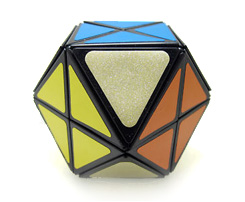Cuboctahedron Twisty Puzzle \$14.95 This "face-turning" twisty puzzle is in the form of a cuboctahedron (eight triangular faces and six square faces).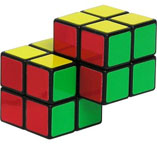Double 2x2 Cube \$9.95 A new twist on on a classic puzzle - a pair of connected 2x2 cubes. On a scale of 1-10, this puzzle rates an 8 for difficulty. 2 1/8 in x 1 1/2 in x 1 1/2 in.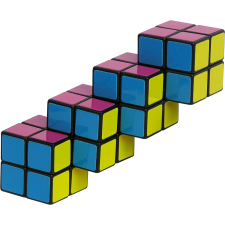Quadruple 2x2 Cube \$14.95 The object of this puzzle is to scramble all of the colors and then restore them to the starting position. This puzzle is extra tricky because it consists of four 2x2 cubes joined together in a row from corner to corner.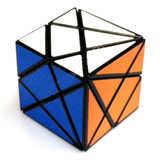Axis Cube \$8.95 This unique twisty puzzle is based on a complex slicing of a cube.